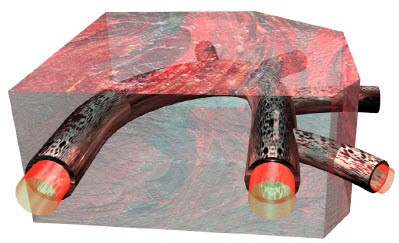# 科技论文和技术资料

#### A Partial Verification of a COMSOL® Example Problem on the Fluid-Structure Interaction in a Network of Blood Vessels

J. T. Fong , A. Heckert , J. J. Filliben 
 National Institute of Standards & Technology, Gaithersburg, MD, USA

Finite element method (FEM)-based solutions are known to contains errors and uncertainties due to at least four mathematical (M) and four physical (P) sources, namely, (M-1) mesh density or degrees of freedom, (M-2) mesh element types, (M-3) mesh quality such as mean aspect ratio, (M-4) FEM platform (P-1) material property coefficients, (P-2) geometric dimensional variabilities, (P-3) initial and boundary loading conditions, and (P-4) boundary constraints. A complete verification of an FEM solution requires an uncertainty quantification of the first four sources, (M-1) through (M-4), and a complete validation, that of the second four sources, (P-1) and (P-4). The goal of this paper is to conduct a partial verification of an example problem in the COMSOL Multiphysics® software on the fluid-structure interaction in a network of blood vessels by applying a nonlinear least square logistic fit method to a sequence of its solutions with increasing degrees of freedom (dof). In other words, our investigation is restricted to the examination of the uncertainty due to one source only, namely (M-1) mesh density, while keeping (M-2) element-type as tetrahedron, (M-3) mesh quality, and (M-4) platform as COMSOL®, fixed. By increasing the degrees of freedom from the coarsest mesh (dof = 159,183) to the finest (def = 679,584), we obtain a sequence of COMSOL® solutions that yield subsets of "plausible" solutions as candidates for a nonlinear least squares logistic fit method to estimate asymptotic solutions with two metrics for accuracy assessment. Our results show that the partially-validated maximum velocity at a specific fixed point in the blood vessel network is 0.49443 mm/s, that is about 11 % higher than the one based on a sequence of coarser meshes (max. vel. = 0.44101 mm/s). The two accuracy metrics are based on the nonlinear least squares logistic fit method with metric-1 based on uncertainty and metric-2, gain in relative error convergence rate.